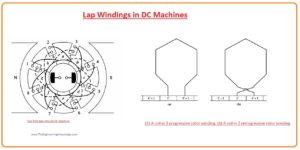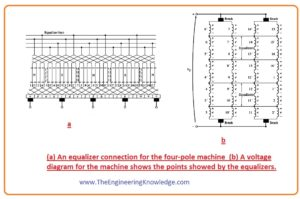Hello, fellows, I hope all of you are having fun in your life. In today’s tutorial, we will discuss the Lap Winding in DC Machines. The rotating part that is the rotor of any dc machine either it is motor or dc generator has a very important part of a machine that is armature of rotor windings. The electrical power transforms in the case of a motor into mechanical and mechanical power into electrical in case of a generator at this part of a machine. There are two main types of rotor windings first one is lap winding and the second one is the wave winding, but there are sub categories of armature windings that are also used like a simple, duplex, multiple, etc.

In today’s post, we will have a detailed look at lap windings, its construction, and connection with the commutator. So let’s get started with the Lap Windings in DC Machines.

#### Lap Winding in DC Machines

• Lap windings is a very simple and normally used winding in dc machines rotors. Simplex lap windings have two ends points and more than one number of turns and its endpoints are connected with neighboring commutators segments.
• If one end of this windings is connected with one commutator segment and second end of windings with other neighboring commutators means if we first end is linked with ‘n’ commutator and second will be connected with ‘n+1’ this type of windings arrangements is known as progressive windings and commutator pitch will be ‘1’ and denoted as yc.
• If the first end of the winding is linked with one commutator segment and other ends with the previous segment, in simple word one end connected with ‘n’ segment and other with ‘n-1’ then this type of winding arrangement is known as retrogressive lap winding. the value of the commutator pitch is ‘-1’.
• The 2 pole dc machine having lap winding is shown in this given figure.• The machine having lap winding have the parallel paths for a current equivalent to the no of poles in the device.
• If we denotes both no of windings and commutators by word ‘C’ and ‘P’ are no of poles then the no of coils in every current path can be fined by the ‘C/P’ formula.
• For a practical understanding of this formula lets discuss this given below figure that has a motor with 4 no of poles.• You can see that there are 4 no of current paths available and the voltage through these paths are equal due to parallel combination.
• The point is that numerous current pathways exist in multiple pole machines that make lap winding the best option less voltage and large current machines, high current does not affect the working of machines having lap windings due to distribution in more than one path.
• This current distribution retains the size of the rotor conductor within certain limits either current has a large value.

#### Problems of Lap Winding in DC Machines

• With the advantage of lap windings in dc machines, there are some drawbacks of lap windings.
• For observation these disadvantages let’s see this given below figure. That has a machine with the 6 no of poles.• Since of extensive working, there is minor wear on the bearings, and the lower conductors of windings are near to poles while upper conductors are at some distance.
• As a consequence, there is a higher value of voltage in the current paths having conductors lower the poles and while current paths having upper conductor have less value of voltage.
• As all current paths are linked with one another in parallel fashion so some parts of the total current come out at the output while some move back in other parts of the machine you can see this in a given figure. 8-17
• It is not perfect for a machine, as the value of resistance at the rotor’s windings has less value, there is a little bit variation in the value of the voltage at parallel paths causes to flow huge current through the brushes that generate a huge amount of heating.

#### How to solve problems of Lap Windings

• The issue occurring due to the circulation of current in the parallel paths of any dc machine having 4 no of poles or larger, cannot be completely reduced but can be minimized to some extent.
• To minimize these problems special windings are used known as
• These equalizers are bars placed the rotor of a machine having lap windings and connected with each other at a similar voltage level at current parallel paths.
• These connection (shorting) of bars allows current to pass through these equalizers instead of brushes of the machines.
• These circulation of current resolves partly flux misbalancing that affected them to exist in the first place.
• An equalizer connected with the machine having 4 poles is shown in given figure.• An equalizer for a large lap dc machine is shown in Figure.• If we wound duplex lap winding on dc machine then there will be 2 complete windings that will be wound on the rotor, each commutator segment will be linked with the single winding.
• So, a discrete coil ends on the 2nd commutator down from where it ongoing, and Yc=+-2.
• As we discussed earlier that every set of windings in lap windings has no parallel paths equal to poles in a machine, so in duplex lap winding the current paths will be twice.
• In simple words for n-plex lap windings, the value of commutator pitch Yc is given as.

Yc=+-n lap windings

• The no of current paths will be.
• a=np
• In this equation.
• a is no of current paths.
• n is plex of windings
• P is no poles.